## 分类问题

• 学习输入到输出的映射 $$f:X\to Y$$
• $$X$$: 输入
• $$Y$$: 输出

## 定义逻辑回归

### 线性回归？

• $$0 \le P(Y|X) \le 1$$
• $$\sum_y P(Y|X) = 1$$

### 逻辑函数？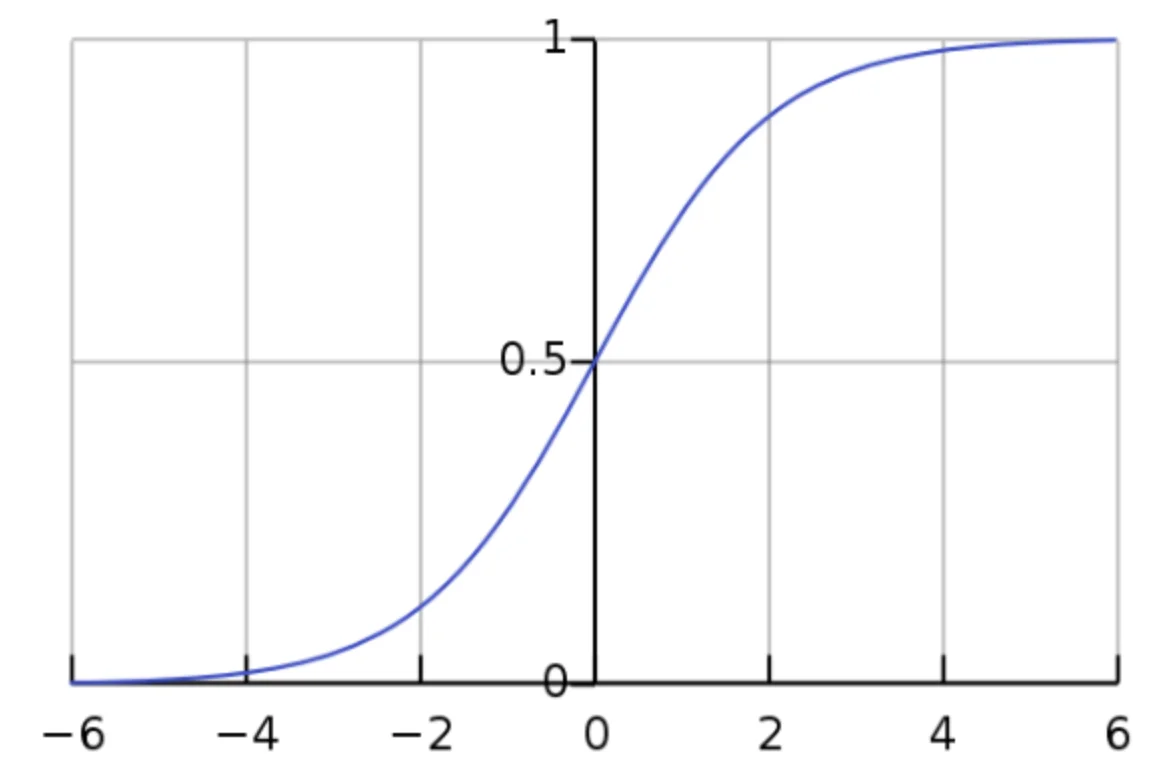• 值域：$$(0,1)$$
• 定义域：$$(-\infty,+\infty)$$
• （从图中可以注意到，当 $$x>6$$ 时，$$y$$ 几乎等于 $$1$$ 了，反之同理。）

### 举个例子

20 4000 本科 YES
25 5000 专科 NO
21 6000 本科 NO
25 5000 专科 YES
28 8000 本科 NO
27 7000 本科

• $$x^{(1)} = \begin{bmatrix} 20 \newline 4000 \newline 本科 \end{bmatrix}$$
• $$w = \begin{bmatrix} w_0 \newline w_1 \newline w_2 \end{bmatrix}$$，（因为有三个参数，所以也需要三个 $$w$$
• $$b \in R$$

## 在求 $$w$$ 和 $$b$$ 前的预备知识

### 逻辑回归的决策边界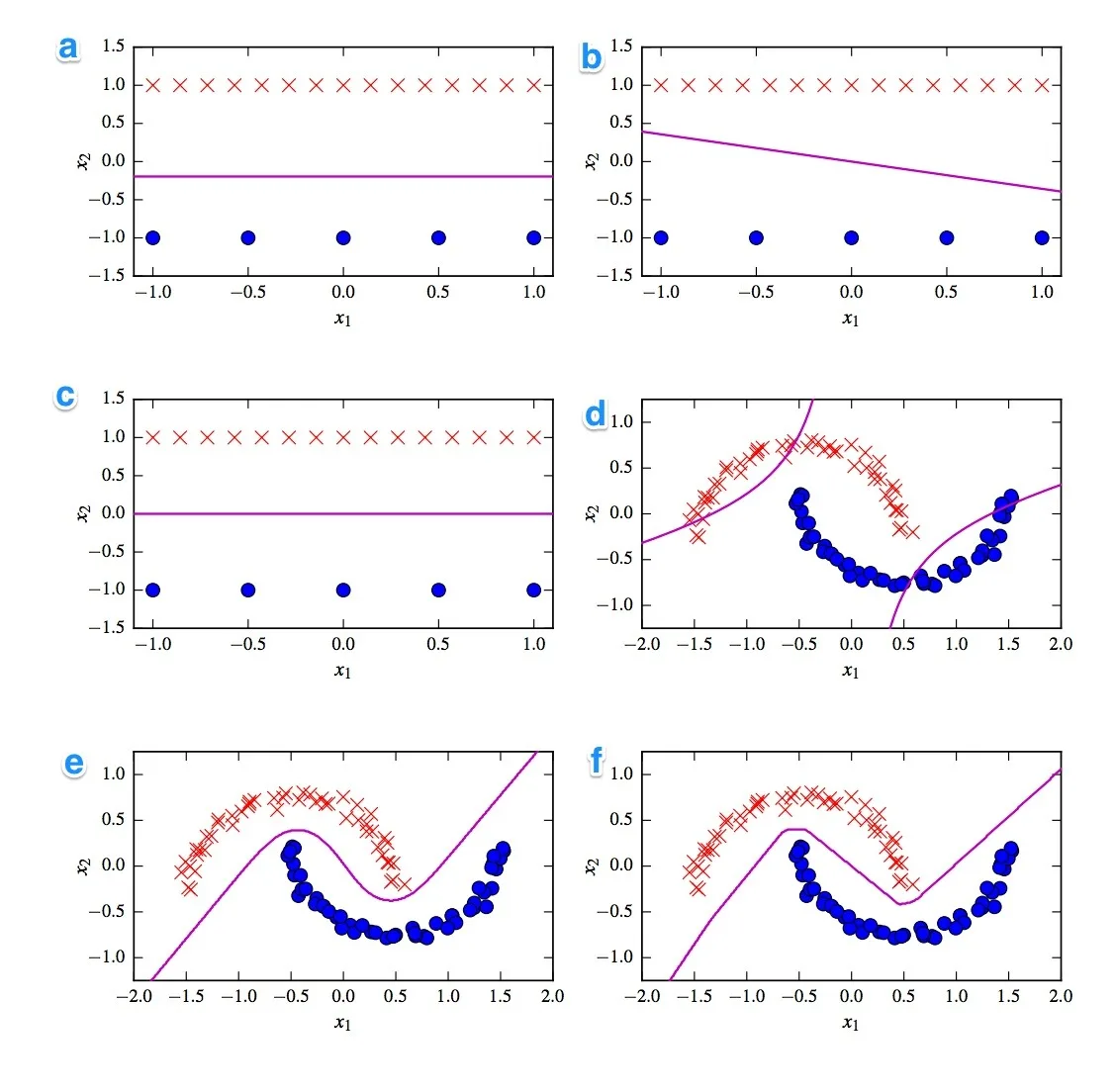### 目标函数

$$w$$$$b$$ 的依据是一个目标函数（Objective Function）。每一个模型都对应着一个目标函数，即我们想最大化/最优化的一个函数。我们再沿着这个函数，找出最优解。

## 求最优的 $$w$$ 和 $$b$$ 的方法

### 凸函数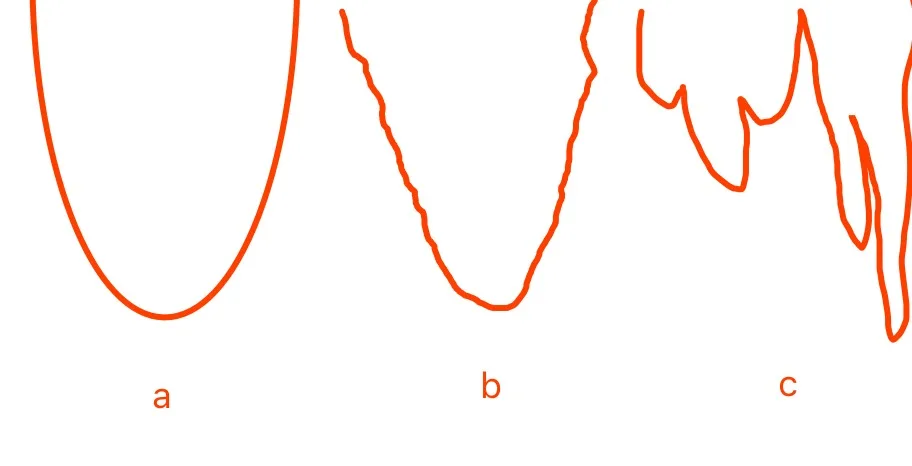b 严格说是不变凸函数（Invex Function），一种凸函数的通型。

#### 凸集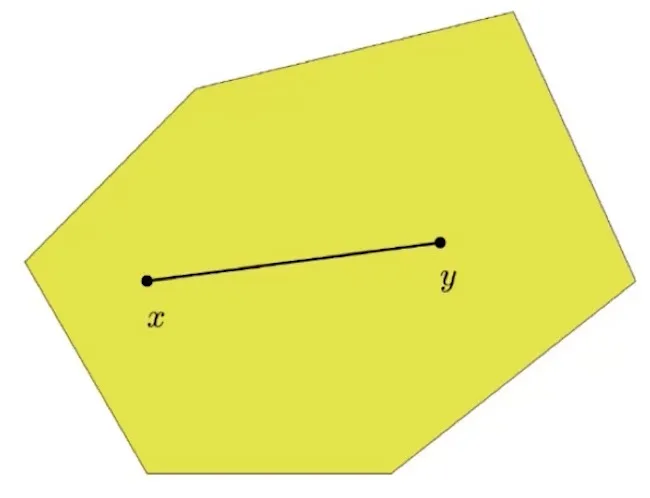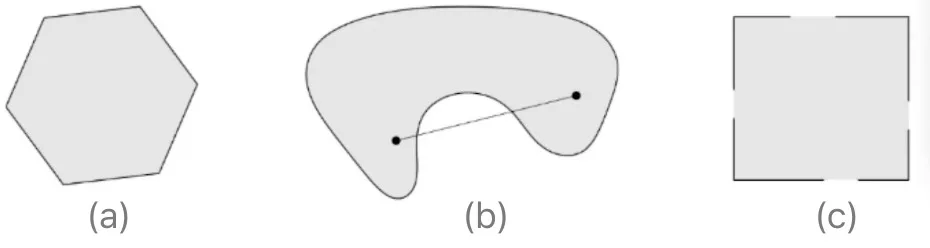• $$R^n$$
• 范数 $$||x|| \le 1$$
• Affine set $$Ax = b$$
• Halfspace $$Ax \le b$$

#### 凸函数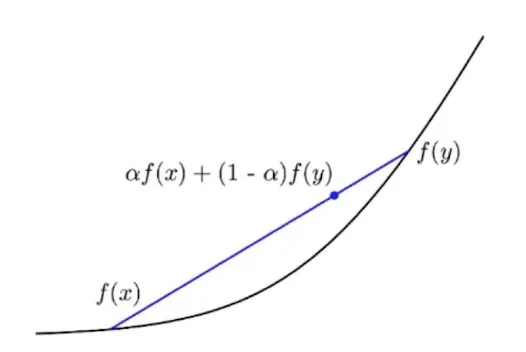• 线性函数是凸/凹函数
• $$e^x, -\log x, x\log x$$
• 范数
• $$\frac{x^Tx}{t}$$

#### 凸函数的判断

##### First Order Convexity Condition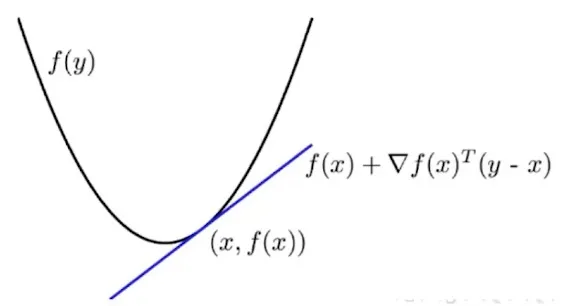## 梯度下降法

### 基本思路

1. 初始化 $$w^1$$

2. $$\text{for}\ t=1,2,3...$$:

$$\ \ w^{t+1} = w^t - \eta \nabla f(w^t)$$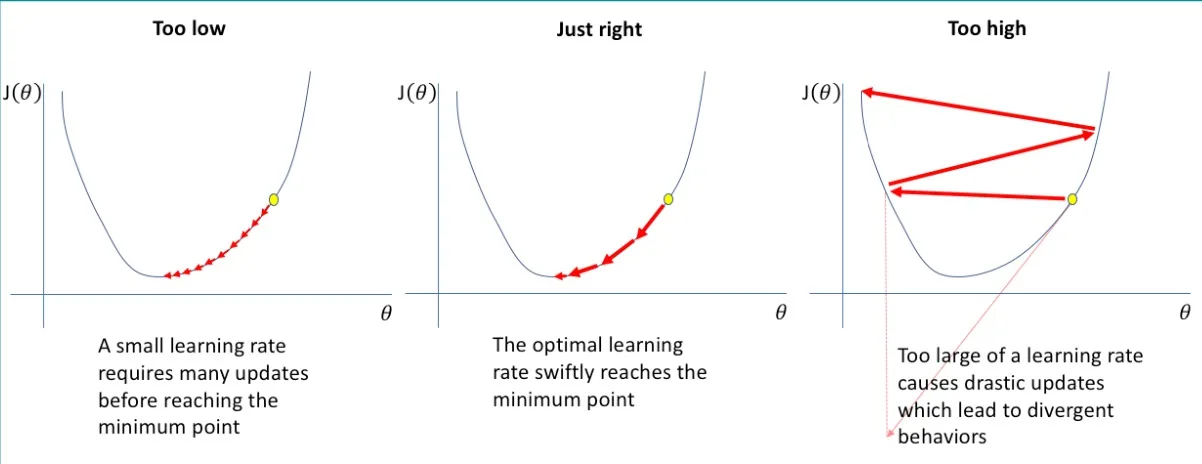### 举个例子

$$f(w) = 4w^2+5w+1$$ 的最优解。

• $$\eta = 0.1$$
• 初始化随机参数：$$w^1 = 0$$, $$f'(w) = 8w+5$$
• $$w^2 = w^1 - 0.1\times(8 \times w^1+5) = -0.5$$
• $$w^3 = w^2 - 0.1\times(8 \times w^2+5) = -0.6$$
• $$w^4 = ... = -0.62$$
• $$w^5 = ... = -0.625$$

### 求逻辑回归的最优解

\begin{align} \frac{\partial L (w,b)}{\partial b} &= - \sum^n_{i=1} y_i \frac{\sigma(w^T x_i + b) [1-\sigma(w^T x_i + b)]}{\sigma(w^T x_i + b)} + (1-y_i) \frac{-\sigma(w^T x_i + b) [1-\sigma(w^T x_i + b)]}{[1 - \sigma(w^T x_i + b)]}\\ &= - \sum^n_{i=1} y_i [1-\sigma(w^T x_i + b)] + (y_i - 1) \sigma(w^T x_i + b) \\ &= - \sum^n_{i=1} [y_i - \sigma(w^T x_i + b)] \\ &= \sum^n_{i=1} [\sigma(w^T x_i + b) - y_i] \end{align}

• 初始化 $$w^1,b^1$$
• $$\text{for}\ t=1,2,3...$$:
• $$w^{t+1} = w^t - \eta \sum ^n_{i=1} [\sigma(w^T x_i + b) - y_i] x_i$$
• $$b^{t+1} = b^t - \eta \sum^n_{i=1} [\sigma(w^T x_i + b) - y_i]$$

1. $$L^t(w,b) - L^{t+1}(w,b) < \epsilon$$
2. $$w^t-w^{t+1}$$ 没有变化
3. Early stopping: validation （验证集的准确性达到要求）
4. Fixed iteration（固定 xx 步内）

### 复杂度分析

#### $$L$$-Lipschitz 介绍

• $$x_k$$: 第 $$k$$ 次迭代的 $$x$$
• $$x^*$$: 最优时的 $$x$$
• $$\frac{\left\|x_{0}-x^{*}\right\|_{2}^{2}}{2 \eta_{t} k}$$: 该项越小，$$x_k$$ 越接近 $$x^*$$。它在迭代中变小得越快，说明这个算法越优质。

#### 收敛性推导

• 第二、七、九步是由 $$x_{i+1} = x_i - \eta_t \nabla f(x_i)$$ 得来的
• 第五步是由 $$\eta_t \le \frac{1}{L}$$ 得来的
• 这个式子 $$f(x_{i+1}) \le f(x_i) - \frac{\eta_t}{2} \|\nabla f(x_i)\|^2$$，由于后一项大于 $$0$$，可以保证 $$f(x_{i+1}) \le f(x_{i})$$，也就是梯度下降法中，新更新的值一直在降低。
• 第六步是由 $$f(y) \geq f(x)+\nabla f(x)^{T}(y-x)$$ 得来的（First Order Convexity Condition）
• 第八步之所以如此转换，是为了得到 $$a^2-2ab+b^2$$ 的模式

## 随机梯度下降法

### 基本思路

• $$\text{for}\ iter=1,2,3,...,T$$
• $$\text{shuffle}(\{x_i,y_i\}_{i=1}^n)$$ （重新排列所有样本）
• $$\text{for}\ i=1,2,3,...,n$$:
• $$w^{new} = w^{old} - \eta [\sigma(w^T x_i + b) - y_i] x_i$$
• $$b^{new} = b^{old} - \eta [\sigma(w^T x_i + b) - y_i]$$

## 过拟合

### 当数据线性可分

If data is linearly separable, then $$w$$ goes to infinity.

\begin{align} P(y = 1 |x,w) &= \frac{1}{1+e^{-(w^T x + b)}} \\ \\ P(y = 0 |x,w) &= 1 - P(y = 1 |x,w) \\ & = \frac{e^{-(w^T x + b)}}{1+e^{-(w^T x + b)}} \end{align}

### 正则化

#### Adding a Term - L2 Norm

$\|w\|_{2}^{2} = w_1^2 + w_2^2+ \cdots + w_n^2$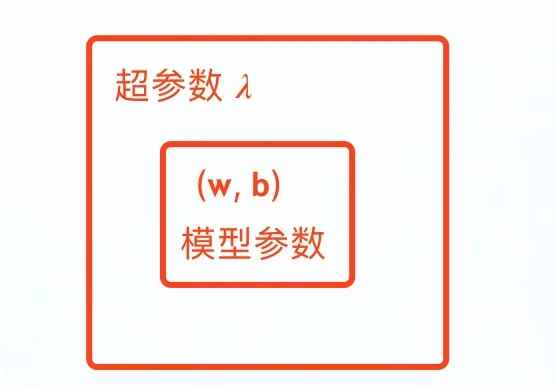\begin{align} \frac{\partial L (w,b) + R(w)}{\partial b} &= \sum^n_{i=1} [\sigma(w^T x_i + b) - y_i] \end{align}

#### 其他范数

Nuclear Norm 可以最小化矩阵的秩（Rank）。

L0 Norm 可以清算向量中非零的数字，即最小化向量中非零数的个数。

#### L1 vs L2

L1 Norm 和 L2 Norm 类似，让 $$w$$ 变小（事实上，L2 Norm 表现更好）。但除此之外，L1 Norm 还有一个特点——让很多 $$w$$ 参数变为 $$0$$，导致稀疏（induces sparse solution）。如果某个参数变为 $$0$$，意味着它事实上对我们的决策没有帮助，可以去掉。所以 L1 Norm 某种程度上也起到一个筛选的作用。当有场景需要筛选一些表现糟糕或无相关的参数时，可以考虑 L1 Norm。

L1 Norm 的一个应用场景是，通过大脑中的神经元激活状态，判断这个人在想什么。我们知道，思考某件事时，只有大脑中一部分区域的神经元在处于活跃。这种情况下，我们会希望采用 L1 Norm 来让参数稀疏，筛选掉无用的神经元参数。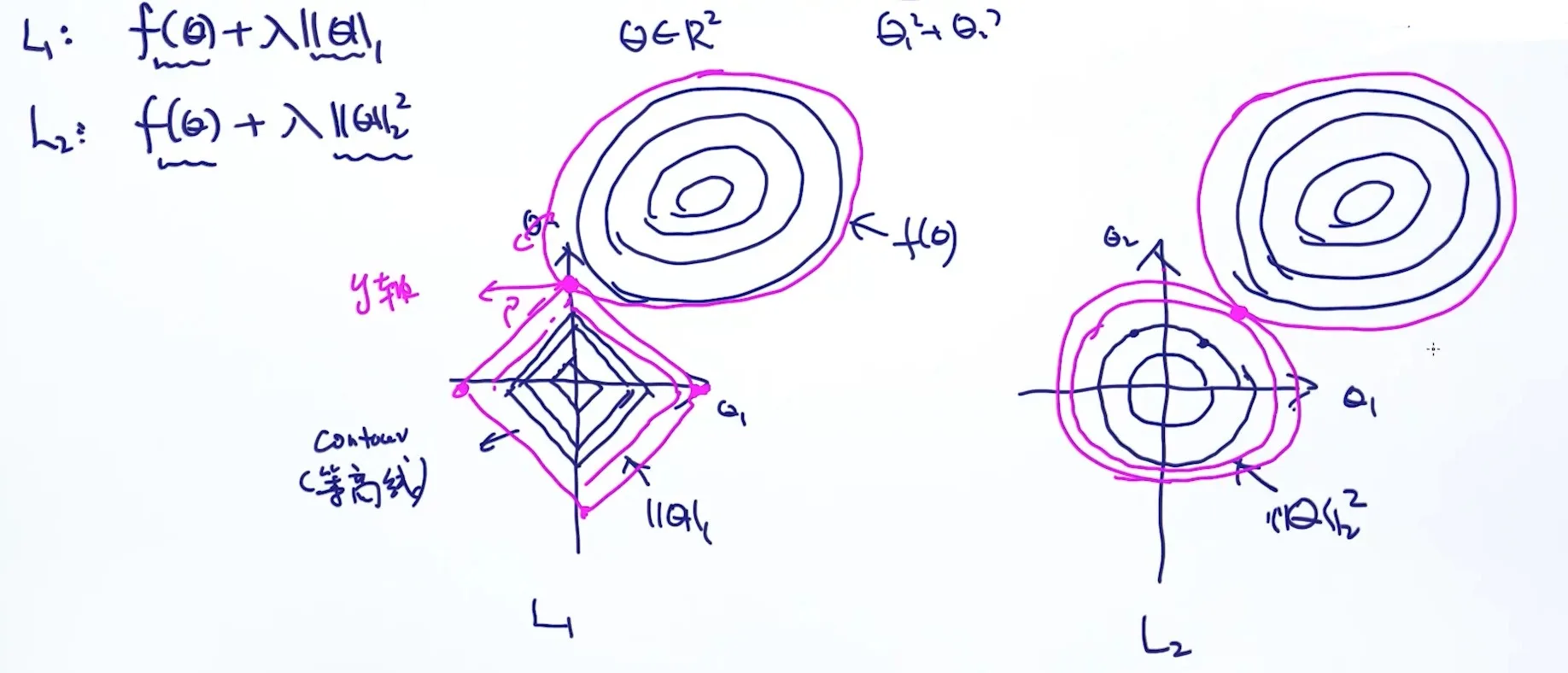#### L1 + L2

• 如果 $$f(w) = \sum_{i=1}^n (w^T x_i + b - y_i) ^2$$
• 那么 $$f(w) + \lambda_2 || w ||_1 + \lambda_2 ||w||_2^2$$ 就叫 Elastic Net。

https://en.wikipedia.org/wiki/Elastic_net_regularization

L1 的另一个问题是，如果某些变量作用类似，我们希望去掉相关性大的「重复作用的」变量。L1 会帮我们随机地在一组类似中选择一项，而不是最好的那一项。但是 Elastic Net 却可以帮我们避免这个问题。为什么呢？请看论文。

### 交叉验证#### K-Fold Cross Validation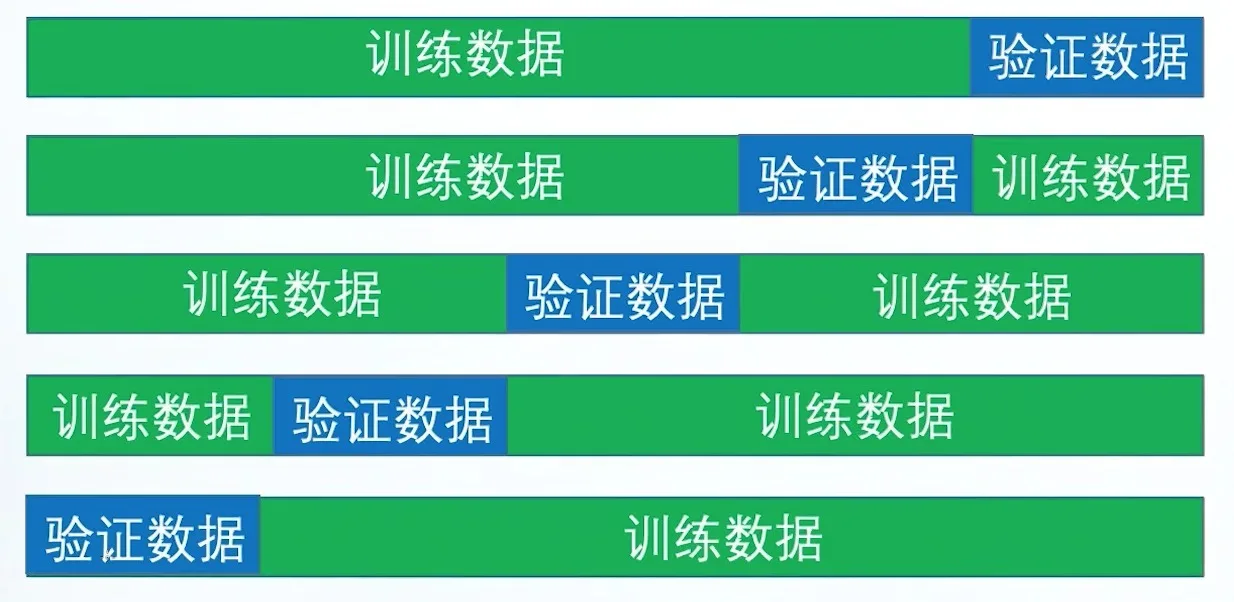• $$\lambda_1 \in (0.001, 0.1)$$
• $$\lambda_2 \in (0.01, 0.5)$$
• 随机选出 $$(\lambda_1, \lambda_2)$$ 的组合

### 模型复杂度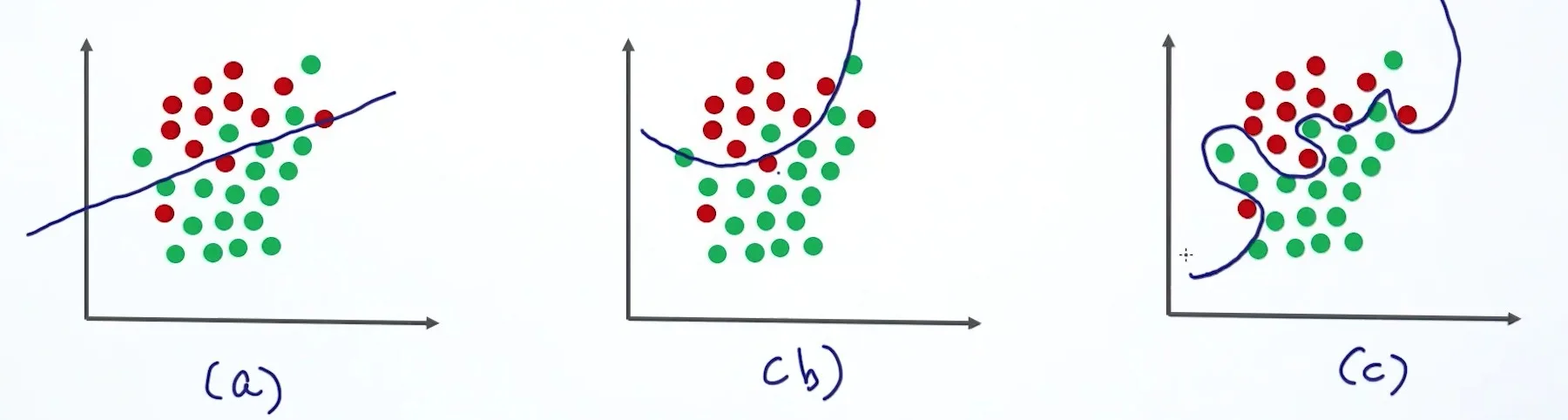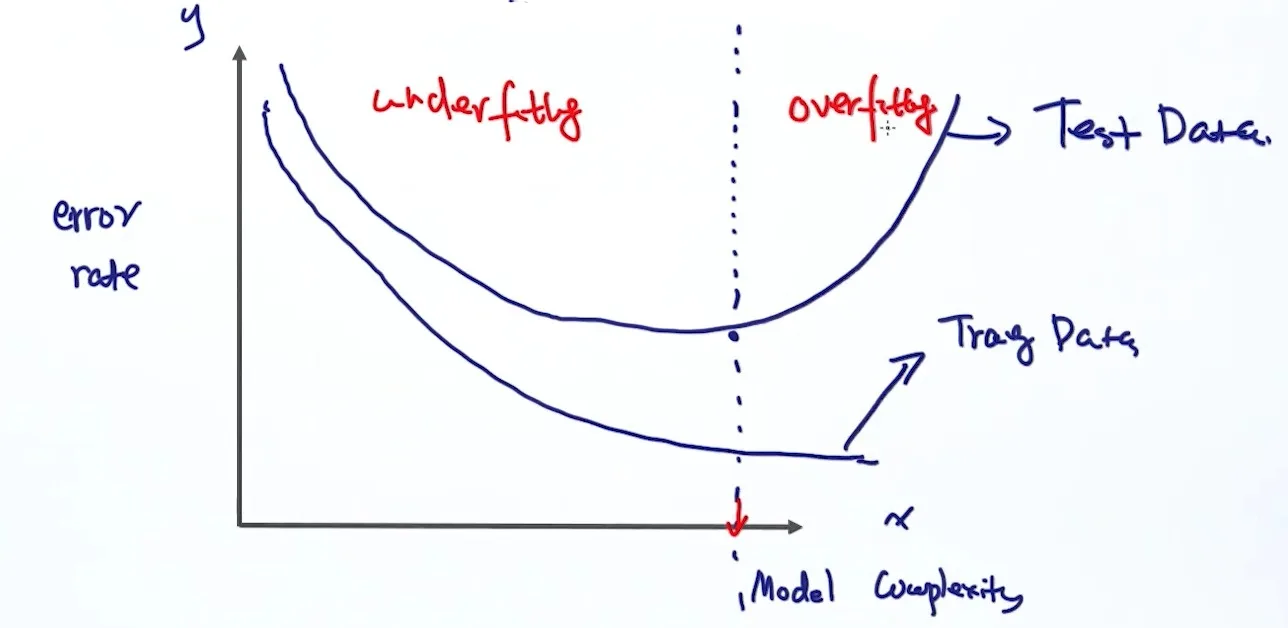#### 影响模型复杂度的因素

##### 模型的种类

• Linner Regression
• Support Vector Machine
• Neural Network
• Deep Learning
• ...